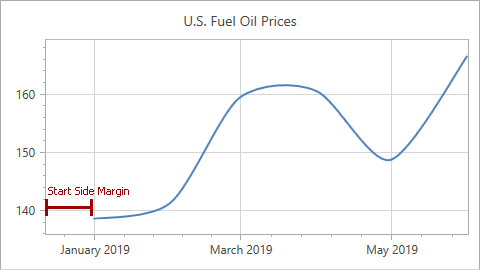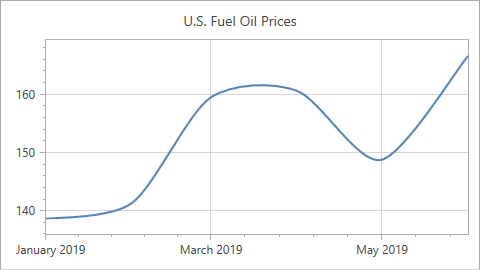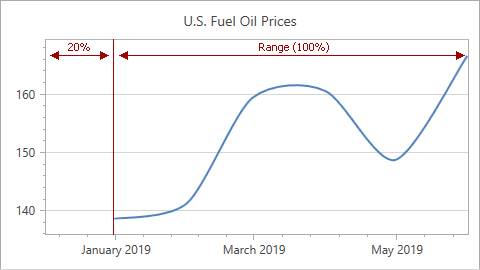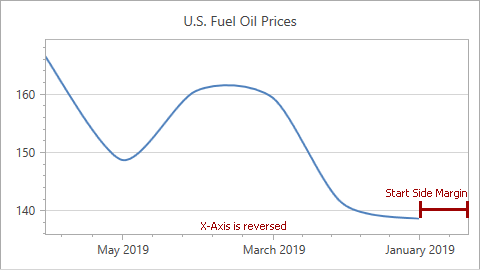# Range.StartSideMargin Property

Gets or sets the distance between the series point with the lowest value and the start of the axis range.

Namespace: DevExpress.Xpf.Charts

Assembly: DevExpress.Xpf.Charts.v21.2.dll

## Declaration

``public double StartSideMargin { get; set; }``

## Property Value

Type Description
Double

The side margin value. SideMarginSizeUnit specifies the unit.

## Remarks

Use an instance of the Range class to access the StartSideMargin property. To specify the margin on the opposite side, use EndSideMargin. Set the Range.SideMarginsValue property to define both side margins.

### Specify Margins in Axis Units

The following images show a chart with different StartSideMargin values (the x-axis measurement unit is Day):

Property Value Example
StartSideMargin = 20 (Days)
(SideMarginSizeUnit = SideMarginSizeUnit.AxisUnit)StartSideMargin = 0 (Days)
(SideMarginSizeUnit = SideMarginSizeUnit.AxisUnit)Markup:

``````<dxc:ChartControl ... >
<dxc:XYDiagram2D>
<dxc:XYDiagram2D.AxisX>
<dxc:AxisX2D ...>
<dxc:AxisX2D.WholeRange>
<dxc:Range StartSideMargin="20"
SideMarginSizeUnit="AxisUnit"/>
</dxc:AxisX2D.WholeRange>
</dxc:AxisX2D>
</dxc:XYDiagram2D.AxisX>
</dxc:XYDiagram2D>
...
</dxc:ChartControl>
``````

At runtime:

``````Range range = new Range();
xAxis.WholeRange = range;
range.SideMarginSizeUnit = SideMarginSizeUnit.AxisUnit;
range.StartSideMargin = 20;
``````

Note: If you set StartSideMargin or EndSideMargin, the control ignores the Range.AutoSideMargins property.

When the SideMarginSizeUnit property is set to AxisUnit, side margins are specified in axis measurement units.

These axis measurement units vary according to the axis type.

Axis Type

Axis Mode

Default Measurement Unit

Date-time

Continuous

DateTimeMeasureUnit.Millisecond

Date-time

Manual, Interval

DateTimeMeasureUnit.Day

Time-span

Continuous

TimeSpanMeasureUnit.Millisecond

Time-span

Manual, Interval

TimeSpanMeasureUnit.Second

Numerical

Continuous, Manual, Interval

Ones

Qualitative

Ones

In Automatic mode, the Chart Control determines an axis measurement unit based on underlying data.

### Specify Margins as a Percentage

Set the Range.SideMarginSizeUnit property to SideMarginSizeUnit.AxisRangePercentage to define a margin value as a percentage of the axis range, for example:Markup:

``````<dxc:ChartControl ... >
<dxc:XYDiagram2D>
<dxc:XYDiagram2D.AxisX>
<dxc:AxisX2D ...>
<dxc:AxisX2D.WholeRange>
<dxc:Range StartSideMargin="20"
SideMarginSizeUnit="AxisRangePercentage"/>
</dxc:AxisX2D.WholeRange>
</dxc:AxisX2D>
</dxc:XYDiagram2D.AxisX>
</dxc:XYDiagram2D>
...
</dxc:ChartControl>
``````

At runtime:

``````Range range = new Range();
xAxis.WholeRange = range;
range.SideMarginSizeUnit = SideMarginSizeUnit.AxisRangePercentage;
range.StartSideMargin = 20;
``````

### Reversed Axis Margins

If the Axis2D.Reverse property is enabled (axis values are arranged in reversed order), side margins change their positions. As a result, the start margin is displayed at the right (for an x-axis) or top (for a y-axis) of the diagram.